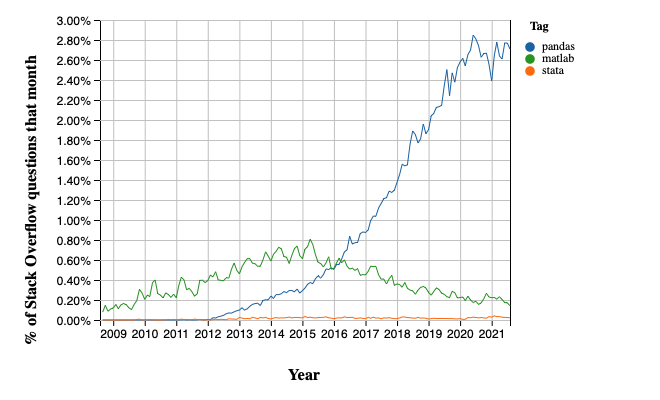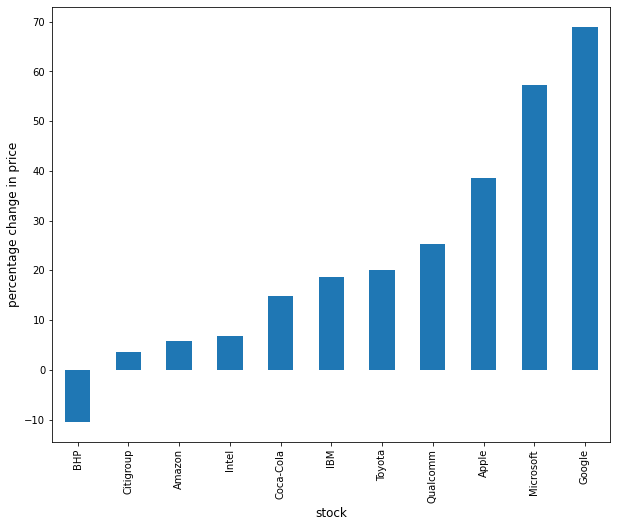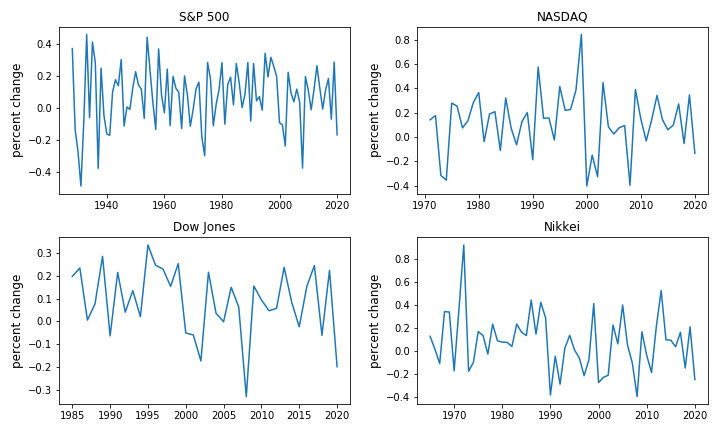# Pandas¶

## Contents¶

In addition to what’s in Anaconda, this lecture will need the following libraries:

In [ ]:
!pip install --upgrade pandas-datareader
!pip install --upgrade yfinance


## Overview¶

Pandas is a package of fast, efficient data analysis tools for Python.

Its popularity has surged in recent years, coincident with the rise of fields such as data science and machine learning.

Here’s a popularity comparison over time against STATA, SAS, and dplyr courtesy of Stack Overflow TrendsJust as NumPy provides the basic array data type plus core array operations, pandas

1. defines fundamental structures for working with data and
2. endows them with methods that facilitate operations such as
• reading in data
• adjusting indices
• working with dates and time series
• sorting, grouping, re-ordering and general data munging 
• dealing with missing values, etc., etc.

More sophisticated statistical functionality is left to other packages, such as statsmodels and scikit-learn, which are built on top of pandas.

This lecture will provide a basic introduction to pandas.

Throughout the lecture, we will assume that the following imports have taken place

In [ ]:
%matplotlib inline
import pandas as pd
import numpy as np
import matplotlib.pyplot as plt
plt.rcParams["figure.figsize"] = [10,8]  # Set default figure size
import requests


## Series¶

Two important data types defined by pandas are Series and DataFrame.

You can think of a Series as a “column” of data, such as a collection of observations on a single variable.

A DataFrame is an object for storing related columns of data.

Let’s start with Series

In [ ]:
s = pd.Series(np.random.randn(4), name='daily returns')
s


Here you can imagine the indices 0, 1, 2, 3 as indexing four listed companies, and the values being daily returns on their shares.

Pandas Series are built on top of NumPy arrays and support many similar operations

In [ ]:
s * 100

In [ ]:
np.abs(s)


But Series provide more than NumPy arrays.

Not only do they have some additional (statistically oriented) methods

In [ ]:
s.describe()


But their indices are more flexible

In [ ]:
s.index = ['AMZN', 'AAPL', 'MSFT', 'GOOG']
s


Viewed in this way, Series are like fast, efficient Python dictionaries (with the restriction that the items in the dictionary all have the same type—in this case, floats).

In fact, you can use much of the same syntax as Python dictionaries

In [ ]:
s['AMZN']

In [ ]:
s['AMZN'] = 0
s

In [ ]:
'AAPL' in s


## DataFrames¶

While a Series is a single column of data, a DataFrame is several columns, one for each variable.

In essence, a DataFrame in pandas is analogous to a (highly optimized) Excel spreadsheet.

Thus, it is a powerful tool for representing and analyzing data that are naturally organized into rows and columns, often with descriptive indexes for individual rows and individual columns.

Here’s the content of test_pwt.csv

"country","country isocode","year","POP","XRAT","tcgdp","cc","cg"
"Argentina","ARG","2000","37335.653","0.9995","295072.21869","75.716805379","5.5788042896"
"Australia","AUS","2000","19053.186","1.72483","541804.6521","67.759025993","6.7200975332"
"India","IND","2000","1006300.297","44.9416","1728144.3748","64.575551328","14.072205773"
"Israel","ISR","2000","6114.57","4.07733","129253.89423","64.436450847","10.266688415"
"Malawi","MWI","2000","11801.505","59.543808333","5026.2217836","74.707624181","11.658954494"
"South Africa","ZAF","2000","45064.098","6.93983","227242.36949","72.718710427","5.7265463933"
"United States","USA","2000","282171.957","1","9898700","72.347054303","6.0324539789"
"Uruguay","URY","2000","3219.793","12.099591667","25255.961693","78.978740282","5.108067988"


Supposing you have this data saved as test_pwt.csv in the present working directory (type %pwd in Jupyter to see what this is), it can be read in as follows:

In [ ]:
df = pd.read_csv('https://raw.githubusercontent.com/QuantEcon/lecture-python-programming/master/source/_static/lecture_specific/pandas/data/test_pwt.csv')
type(df)

In [ ]:
df


We can select particular rows using standard Python array slicing notation

In [ ]:
df[2:5]


To select columns, we can pass a list containing the names of the desired columns represented as strings

In [ ]:
df[['country', 'tcgdp']]


To select both rows and columns using integers, the iloc attribute should be used with the format .iloc[rows, columns]

In [ ]:
df.iloc[2:5, 0:4]


To select rows and columns using a mixture of integers and labels, the loc attribute can be used in a similar way

In [ ]:
df.loc[df.index[2:5], ['country', 'tcgdp']]


Let’s imagine that we’re only interested in population (POP) and total GDP (tcgdp).

One way to strip the data frame df down to only these variables is to overwrite the dataframe using the selection method described above

In [ ]:
df = df[['country', 'POP', 'tcgdp']]
df


Here the index 0, 1,..., 7 is redundant because we can use the country names as an index.

To do this, we set the index to be the country variable in the dataframe

In [ ]:
df = df.set_index('country')
df


Let’s give the columns slightly better names

In [ ]:
df.columns = 'population', 'total GDP'
df


Population is in thousands, let’s revert to single units

In [ ]:
df['population'] = df['population'] * 1e3
df


Next, we’re going to add a column showing real GDP per capita, multiplying by 1,000,000 as we go because total GDP is in millions

In [ ]:
df['GDP percap'] = df['total GDP'] * 1e6 / df['population']
df


One of the nice things about pandas DataFrame and Series objects is that they have methods for plotting and visualization that work through Matplotlib.

For example, we can easily generate a bar plot of GDP per capita

In [ ]:
ax = df['GDP percap'].plot(kind='bar')
ax.set_xlabel('country', fontsize=12)
ax.set_ylabel('GDP per capita', fontsize=12)
plt.show()


At the moment the data frame is ordered alphabetically on the countries—let’s change it to GDP per capita

In [ ]:
df = df.sort_values(by='GDP percap', ascending=False)
df


Plotting as before now yields

In [ ]:
ax = df['GDP percap'].plot(kind='bar')
ax.set_xlabel('country', fontsize=12)
ax.set_ylabel('GDP per capita', fontsize=12)
plt.show()


## On-Line Data Sources¶

Python makes it straightforward to query online databases programmatically.

An important database for economists is FRED — a vast collection of time series data maintained by the St. Louis Fed.

For example, suppose that we are interested in the unemployment rate.

Via FRED, the entire series for the US civilian unemployment rate can be downloaded directly by entering this URL into your browser (note that this requires an internet connection)

https://research.stlouisfed.org/fred2/series/UNRATE/downloaddata/UNRATE.csv


(Equivalently, click here: https://research.stlouisfed.org/fred2/series/UNRATE/downloaddata/UNRATE.csv)

This request returns a CSV file, which will be handled by your default application for this class of files.

Alternatively, we can access the CSV file from within a Python program.

This can be done with a variety of methods.

We start with a relatively low-level method and then return to pandas.

### Accessing Data with requests¶

One option is to use requests, a standard Python library for requesting data over the Internet.

To begin, try the following code on your computer

In [ ]:
r = requests.get('http://research.stlouisfed.org/fred2/series/UNRATE/downloaddata/UNRATE.csv')


If there’s no error message, then the call has succeeded.

If you do get an error, then there are two likely causes

1. You are not connected to the Internet — hopefully, this isn’t the case.
2. Your machine is accessing the Internet through a proxy server, and Python isn’t aware of this.

In the second case, you can either

Assuming that all is working, you can now proceed to use the source object returned by the call requests.get('http://research.stlouisfed.org/fred2/series/UNRATE/downloaddata/UNRATE.csv')

In [ ]:
url = 'http://research.stlouisfed.org/fred2/series/UNRATE/downloaddata/UNRATE.csv'
source = requests.get(url).content.decode().split("\n")
source

In [ ]:
source

In [ ]:
source


We could now write some additional code to parse this text and store it as an array.

But this is unnecessary — pandas’ read_csv function can handle the task for us.

We use parse_dates=True so that pandas recognizes our dates column, allowing for simple date filtering

In [ ]:
data = pd.read_csv(url, index_col=0, parse_dates=True)


The data has been read into a pandas DataFrame called data that we can now manipulate in the usual way

In [ ]:
type(data)

In [ ]:
data.head()  # A useful method to get a quick look at a data frame

In [ ]:
pd.set_option('precision', 1)
data.describe()  # Your output might differ slightly


We can also plot the unemployment rate from 2006 to 2012 as follows

In [ ]:
ax = data['2006':'2012'].plot(title='US Unemployment Rate', legend=False)
ax.set_xlabel('year', fontsize=12)
ax.set_ylabel('%', fontsize=12)
plt.show()


Note that pandas offers many other file type alternatives.

Pandas has a wide variety of top-level methods that we can use to read, excel, json, parquet or plug straight into a database server.

### Using pandas_datareader and yfinance to Access Data¶

The maker of pandas has also authored a library called pandas_datareader that gives programmatic access to many data sources straight from the Jupyter notebook.

While some sources require an access key, many of the most important (e.g., FRED, OECD, EUROSTAT and the World Bank) are free to use.

We will also use yfinance to fetch data from Yahoo finance in the exercises.

For now let’s work through one example of downloading and plotting data — this time from the World Bank.

Note

There are also other python libraries available for working with world bank data such as wbgapi

The World Bank collects and organizes data on a huge range of indicators.

For example, here’s some data on government debt as a ratio to GDP.

The next code example fetches the data for you and plots time series for the US and Australia

In [ ]:
from pandas_datareader import wb

govt_debt = wb.download(indicator='GC.DOD.TOTL.GD.ZS', country=['US', 'AU'], start=2005, end=2016).stack().unstack(0)
ind = govt_debt.index.droplevel(-1)
govt_debt.index = ind
ax = govt_debt.plot(lw=2)
ax.set_xlabel('year', fontsize=12)
plt.title("Government Debt to GDP (%)")
plt.show()


The documentation provides more details on how to access various data sources.

## Exercises¶

### Exercise 1¶

With these imports:

In [ ]:
import datetime as dt
import yfinance as yf


Write a program to calculate the percentage price change over 2019 for the following shares:

In [ ]:
ticker_list = {'INTC': 'Intel',
'MSFT': 'Microsoft',
'IBM': 'IBM',
'BHP': 'BHP',
'TM': 'Toyota',
'AAPL': 'Apple',
'AMZN': 'Amazon',
'BA': 'Boeing',
'QCOM': 'Qualcomm',
'KO': 'Coca-Cola',
'GOOG': 'Google',
'SNE': 'Sony',
'PTR': 'PetroChina'}


Here’s the first part of the program

In [ ]:
def read_data(ticker_list,
start=dt.datetime(2019, 1, 2),
end=dt.datetime(2019, 12, 31)):
"""
This function reads in closing price data from Yahoo
for each tick in the ticker_list.
"""
ticker = pd.DataFrame()

for tick in ticker_list:
stock = yf.Ticker(tick)
prices = stock.history(start=start, end=end)
closing_prices = prices['Close']
ticker[tick] = closing_prices

return ticker

ticker = read_data(ticker_list)


Complete the program to plot the result as a bar graph like this one:### Exercise 2¶

Using the method read_data introduced in Exercise 1, write a program to obtain year-on-year percentage change for the following indices:

In [ ]:
indices_list = {'^GSPC': 'S&P 500',
'^IXIC': 'NASDAQ',
'^DJI': 'Dow Jones',
'^N225': 'Nikkei'}


Complete the program to show summary statistics and plot the result as a time series graph like this one:## Solutions¶

### Exercise 1¶

There are a few ways to approach this problem using Pandas to calculate the percentage change.

First, you can extract the data and perform the calculation such as:

In [ ]:
p1 = ticker.iloc    #Get the first set of prices as a Series
p2 = ticker.iloc[-1]   #Get the last set of prices as a Series
price_change = (p2 - p1) / p1 * 100
price_change


Alternatively you can use an inbuilt method pct_change and configure it to perform the correct calculation using periods argument.

In [ ]:
change = ticker.pct_change(periods=len(ticker)-1, axis='rows')*100
price_change = change.iloc[-1]
price_change


Then to plot the chart

In [ ]:
price_change.sort_values(inplace=True)
price_change = price_change.rename(index=ticker_list)
fig, ax = plt.subplots(figsize=(10,8))
ax.set_xlabel('stock', fontsize=12)
ax.set_ylabel('percentage change in price', fontsize=12)
price_change.plot(kind='bar', ax=ax)
plt.show()


### Exercise 2¶

Following the work you did in Exercise 1, you can query the data using read_data by updating the start and end dates accordingly.

In [ ]:
indices_data = read_data(
indices_list,
start=dt.datetime(1971, 1, 1),  #Common Start Date
end=dt.datetime(2020, 12, 31)
)


Then, extract the first and last set of prices per year as DataFrames and calculate the yearly returns such as:

In [ ]:
yearly_returns = pd.DataFrame()

for index, name in indices_list.items():
p1 = indices_data.groupby(indices_data.index.year)[index].first()  # Get the first set of returns as a DataFrame
p2 = indices_data.groupby(indices_data.index.year)[index].last()   # Get the last set of returns as a DataFrame
returns = (p2 - p1) / p1
yearly_returns[name] = returns

yearly_returns


Next, you can obtain summary statistics by using the method describe.

In [ ]:
yearly_returns.describe()


Then, to plot the chart

In [ ]:
fig, axes = plt.subplots(2, 2, figsize=(10, 8))

for iter_, ax in enumerate(axes.flatten()):            # Flatten 2-D array to 1-D array
index_name = yearly_returns.columns[iter_]         # Get index name per iteration
ax.plot(yearly_returns[index_name])                # Plot pct change of yearly returns per index
ax.set_ylabel("percent change", fontsize = 12)
ax.set_title(index_name)

plt.tight_layout()


 Wikipedia defines munging as cleaning data from one raw form into a structured, purged one.